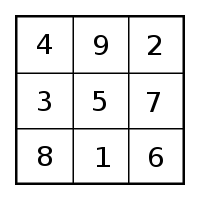Number Square Standard Set by Sparta/AthensNumber Square Standard Set by Sparta/Athens. There are two types of number squares. First are the odd numbered. Sparta puts forth the odd ideals.  This is set in the 1st number square. It is the 3 x 3. The second type are the even. Athens was the prototype of the 4 x 4 number square. It is the 1st even number square. This blog will concern itself only with centers of these 1st two squares in this infinite series.  As described by Hesiod, peace and plenty once marked  a lost Golden Age. It was maintained by these number squares.  Below are couple of internal links. They can offer an idea of  how they were used.Ideals for Sparta were rigid and military

Number Square Standard of Sparta/Athens Identified

Here is a quick review of some basic ideals of each of these two city-states and the number square standard.Interconnection and love are demonstrated by the 4 numbers that interact at the center.Independence and strength come from the center of this prime example.

So What About the Number Square Centers for Sparta and Athens?

• In Sparta, military used rank, order, rigidity in a caste system. Thus the center of the 3 x 3 number square seemingly stands alone. It is marked by number “5”. It does have interconnections. However, they are there but lie  hidden.They have been waiting for a Golden Age to be uncovered.
• In Athens both culture and philosophy, as is true everywhere, are based on interconnection. Here’s how this parallels the 4 x 4 square. : Note the 4 numbers at the center of the 4 x 4 square. Set one consists of  6 + 11 = 17. Set two has  10 + 7 = 17. Here we have a central equality by addition. The equality exists in a second manner way by multiplication: Take the same two pairs of numbers. This time, multiply them as ( 6 x 11)  and  (10 x 7).  As 66 + 70, they total  136. Next,  total the square’s individual numbers from 1 thru 16. They total also 136. This means the full numerical potential of the square exists in this cross multiplication and then addition of the four central numbers in the manner described. This sets the pattern for all even number squares.

Conclusion: These two city states were conceived to represent the two aspects of a lost number square order. They graphically illustrate the basic concepts behind both types of centers. The 1st chapter of Genesis accomplishes exactly the same. It has also preserved this lost order for the future. The time is here to revive this happy and even joyful, lost epoch. My education and understanding of number squares came from a female spirit belonging to  the Lennie Lenape.  The Oquaga Spirit Speaks is such a product on DSOworks.com. Click on the link below.